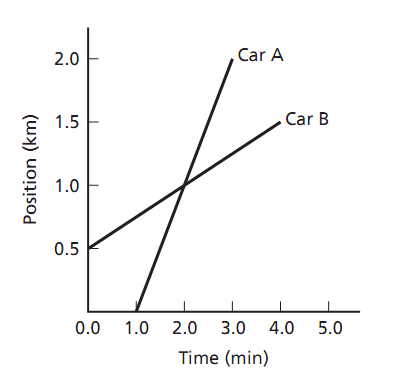## At what time do the cars meet, Physics

Assignment Help:

Two cars head out in the similar direction. Car A starts 1.0 min before car B. The position-time graphs for both cars are shown in the accompanying figure.a. How far apart are the two cars when car B starts out at t = 1.0 min?

0.75 km

b. At what time do the cars meet?

2.0 min

c. How far apart are the cars at time t = 3.0 min?

0.75 km

electric field

#### Anekardhi shabdh, list of anekardhi shabdh with examples

list of anekardhi shabdh with examples

#### Single pv cell design., give the complete explaination with design formulae...

give the complete explaination with design formulae of single pv cell?

#### Define atmospheric energy - thermodynamics, Define Atmospheric Energy - the...

Define Atmospheric Energy - thermodynamics? The mass of the Earth's atmosphere, p(z), varies with height z above the Earth's surface. Assume that the "thickness" of the atmosp

#### Thermal energy, the sun warming the earth is an example of?

the sun warming the earth is an example of?

#### What is deforming force, If a force applied on a body causes a alteration i...

If a force applied on a body causes a alteration in the normal positions of the molecules of the body, resulting in a alteration in the configuration of the object either in length

#### Derive the Electric Field, Start from the expression of the electric field ...

Start from the expression of the electric field outside an infinite sheet of uniform surface charge density, E = s/2epsilon, derive the electric field just outside an ideal conduct

#### What is efficiency of a transformer, Q. What is efficiency of a transformer...

Q. What is efficiency of a transformer? Efficiency of a transformer is described as the ratio of output power to the input power. η= output power / input power

#### Why does the electric field inside a dielectric decrease, Normal 0 ...

Normal 0 false false false EN-IN X-NONE X-NONE MicrosoftInternetExplorer4

#### Determine phase of vibration of two vibrating particles, Two vibrating part...

Two vibrating particles that are "out of phase" differ in the phase of their vibration by: a) 1/4 cycle b) 1/2 cycle c) 3/4 cycle d) 1 cycle Ans: Two vibra